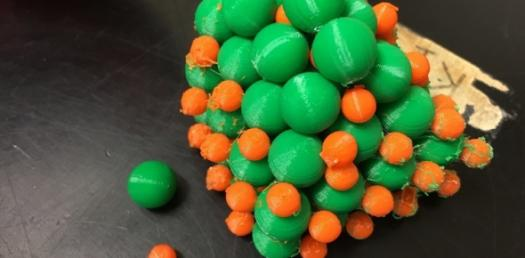# Ionic Bond And Ionic Compounds! Trivia Questions Quiz

25 Questions | Attempts: 184SettingsAre you a chemistry student looking to test your understanding on Ionic Bond and Ionic Compounds? These types of bonds are what keep atoms together during the formation of compounds. Do take up the quiz and get to see if you know how to write formulas of specific compounds. All the best and keep a look out for other quizzes like it!

• 1.
What is the formula for Magnesium Oxide?
• 2.
What is the formula for Aluminum Nitride?
• 3.
What is the formula for Lithium Iodide?
• 4.
What is the formula for Sodium Fluoride?
• 5.
What is the formula for Beryllium Oxide?
• 6.
What is the name of the compound AlCl3?
• 7.
What is the name of the compound Mg3N2?
• 8.
What is the name of the compound CaO?
• 9.
What is the name of the compound MgI2?
• 10.
What is the name of the compound NiO?
• 11.
What is the name of the compound NaBr?
• 12.
What is the name of the compound KCl?
• 13.
What is the formula for Mercury II Oxide?
• 14.
What is the formula for Lithium Sulfide?
• 15.
Which of the following types of ions is positive?
• A.

Cation

• B.

Anion

• 16.
Which of the following ions is negative?
• A.

Cation

• B.

Anion

• 17.
Which of the following are properties of ionic compounds
• A.

Low melting temperature

• B.

High boiling point

• C.

Hard

• D.

Brittle

• E.

Electrolyte in water

• F.

Does not conduct electricity in water

• G.

Does not conduct electricity as a solid.

• 18.
The goal of all atoms is to become _____
• 19.
Which will have more lattice energy?
• A.

LiF

• B.

KBr

• C.

NaCl

• D.

RbI

• 20.
Which would have more lattice energy?
• A.

K2S

• B.

CaO

• C.

GaN

• D.

AlCl3

• 21.
How many Valence Electrons does Chlorine have?
• 22.
What is Zinc's oxidation number ALWAYS?
• 23.
What is Sodium's Oxidation number?
• 24.
When drawing lewis structures what do all of the ions need to have around it?
• A.

Brackets

• B.

Charges

• C.

Full Valence Shells

• D.

The center atom always having 8 VE

• 25.
What is the symbol for Potassium
• A.

K

• B.

P

• C.

Po

• D.

Na

## Related TopicsBack to top
×

Wait!
Here's an interesting quiz for you.